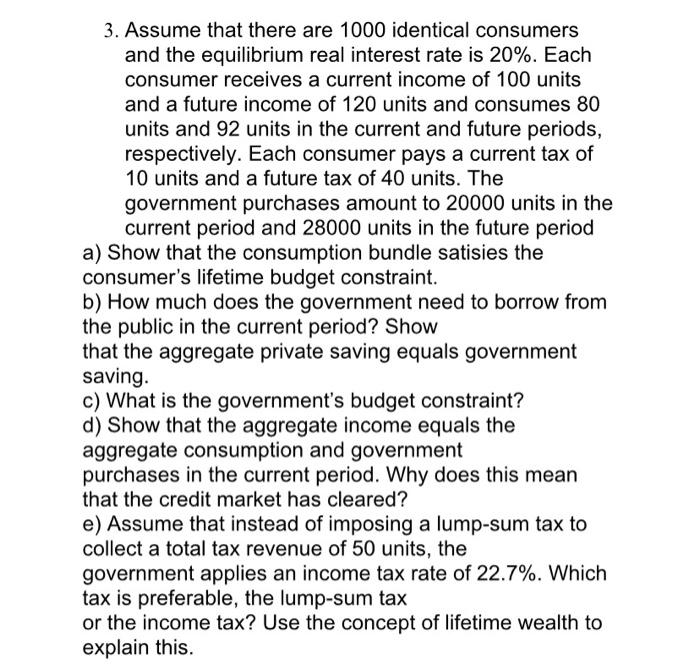Home / Expert Answers / Economics / 3-assume-that-there-are-1000-identical-consumers-and-the-equilibrium-real-interest-rate-is-20-pa597

# (Solved): 3. Assume that there are 1000 identical consumers and the equilibrium real interest rate is \( 20 \ ...3. Assume that there are 1000 identical consumers and the equilibrium real interest rate is \( 20 \% \). Each consumer receives a current income of 100 units and a future income of 120 units and consumes 80 units and 92 units in the current and future periods, respectively. Each consumer pays a current tax of 10 units and a future tax of 40 units. The government purchases amount to 20000 units in the current period and 28000 units in the future period a) Show that the consumption bundle satisies the consumer's lifetime budget constraint. b) How much does the government need to borrow from the public in the current period? Show that the aggregate private saving equals government saving. c) What is the government's budget constraint? d) Show that the aggregate income equals the aggregate consumption and government purchases in the current period. Why does this mean that the credit market has cleared? e) Assume that instead of imposing a lump-sum tax to collect a total tax revenue of 50 units, the government applies an income tax rate of \( 22.7 \% \). Which tax is preferable, the lump-sum tax or the income tax? Use the concept of lifetime wealth to explain this.

We have an Answer from Expert